# Understanding Stabilization Methods

May 30, 2014

Most numerical simulation methods (finite elements, finite volumes, and finite differences) require stabilization methods when modeling transport applications driven mainly by convection rather than diffusion. With the finite element method (FEM), stabilization means adding a small amount of artificial diffusion. This leads to more robust and faster computational performance. Here, we provide insight on the impact of stabilization on your numerical model. We also look at an alternative numerical method that is very efficient and does not require any stabilization.

### When Do We Need Stabilization?

COMSOL Multiphysics uses the finite element method (FEM), which is a well-known and established approach to solving the governing Partial Differential Equations (PDEs) numerically. When solving solid mechanics applications or transport phenomena driven by diffusion, the approach is straight-forward. For convection-dominated transport problems, however, it is known that the approach can lead to numerical instabilities, namely, oscillations in the solution. COMSOL Multiphysics automatically uses stabilization methods to prevent this phenomenon. Nevertheless, some knowledge can be really helpful to understand the performance of transport simulations.

Let’s start with a general convection-diffusion transport equation for an unknown solution, u:

\frac{\partial u}{\partial t}+\beta\cdot \nabla u = \nabla\cdot(c\nabla u)+F

The parameters \beta and c refer to the convective velocity vector and the diffusion coefficient, respectively, while F represents an arbitrary source term. This equation could represent the energy equation, i.e., the heat transfer equation, mass transport equations (used for the transport of chemical species), Navier-Stokes equations for the transport of momentum in fluids, or any other transport equation. For a uniform mesh with first-order shape functions, it has been mathematically proven that numerical instabilities occur when the element Péclet number \mathrm{Pe} exceeds 1:

\mathrm{Pe}:=\frac{\|\beta\|h}{2c}>1

with h being the mesh element size.

The element Péclet number relates the convective and diffusive effects. Either large convective or small diffusive activity leads to a Péclet number larger than 1. But this is only half-true, since the mesh element size plays an important role, too. The higher the mesh resolution, the smaller the element Péclet number. This also means that for every non-zero diffusion term, there exists a mesh resolution that forces the element Péclet number to a value smaller than 1 in the whole computational domain.

However, such a mesh can be computationally expensive or even unfeasible. Stabilization methods allow for simulation on coarser meshes, thus drastically reducing the computational load.

### A Mass Transport Example

To better understand the effect of stabilization methods, let’s look at an example and solve it with COMSOL Multiphysics. We consider a time-dependent mass transfer problem. Let’s imagine we have a three meter long pipe with a plug flow (a flow with constant velocity across the pipe cross section) of water. The velocity of the plug flow is 1 m/s. At the beginning of the experiment (i.e., at t = 0), a chemical species is dissolved in the water with the following concentration: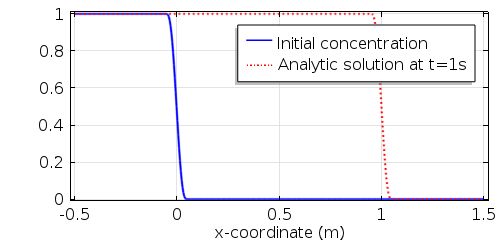The initial concentration (in blue) is set to 1 for x 1 m. This plot also shows the analytic solution for the concentration at t = 1 s. Because the fluid velocity is equal to 1 m/s, the concentration discontinuity should be located at x = 1 m. Next, our goal is to find the evolution of the concentration profile as a function of time.

We can model this problem in 1D in COMSOL Multiphysics using the Transport of Dilute Species physics interface. In this physics interface, we compute the evolution in time of the chemical species concentration, driven by convection and diffusion. The velocity is set to \beta=1~\mathrm{m/s} and the diffusion coefficient to c=10^{-9} ~\mathrm{m^2/s}.

At the inlet, we prescribe a concentration of 1~\mathrm{mol/m^3}. A mesh size, \Delta x=0.05, results in element Péclet numbers around 25 \cdot106, which is far beyond the critical value of 1. After running the time-dependent solver up to t = 1 s, the following solution (solid blue line) would be obtained without stabilization. For comparison, we also show the analytic solution (dotted red line).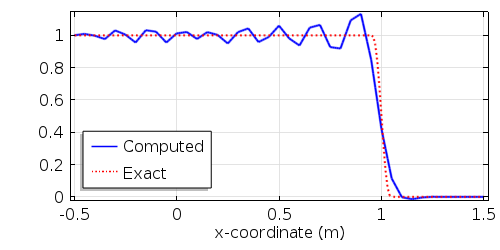We clearly see that the computed solution is useless. Oscillations are not only present around the concentration gradients, but are spread over the whole domain. These oscillations can occur where the Péclet number exceeds one and any of the following conditions exist:

1. A space-dependent initial condition, which the mesh does not resolve
2. A Dirichlet boundary condition leading to a solution containing a steep gradient near the boundary and forming a boundary layer
3. A small initial diffusion term close to a non-constant source term or a non-constant Dirichlet boundary condition

This mass transport example illustrates the first point, since we chose a space-dependent initial concentration profile. We can determine the mesh element size that forces the Péclet number to be smaller than 1:

h\leq \frac{2c}{\|\beta\|}=2\cdot10^{-9}

Such a small mesh element size for the whole domain would result in more than 1 billion elements. This should be motivation enough to consider a different option.

### Stabilization with COMSOL Multiphysics

All transport interfaces, such as heat transfer, fluid flow, or species transport, automatically use stabilization. In order to see the corresponding settings, you first need to switch on “Stabilization” by clicking the eye symbol above the Model Builder. In the physics node, you will then find two additional sections, namely “Consistent Stabilization” and “Inconsistent Stabilization”, as shown in the screenshot for the Transport of Diluted Species interface:How to make the stabilization settings visible.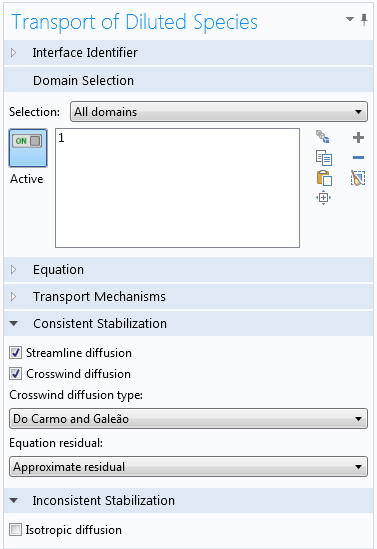Stabilization settings in the Transport of Diluted Species interface.

By default, consistent stabilization is checked, because this method works well for most applications.

In the following section, we will briefly explain the idea of both methods and show the corresponding results for the mass transport example. The main idea of all stabilization methods is to add additional diffusive terms, in order to decrease the Péclet number. As simple as this sounds, this introduction of additional diffusion can be realized in many different ways.

#### Inconsistent Stabilization: Isotropic Diffusion

The most simple approach is to define an artificial diffusion coefficient, c_{\mathrm{art}}, as:

c_{\mathrm{art}}=\delta h\|\beta\|

and add it to the physical diffusion coefficient, c, giving an overall diffusion of c+c_{\mathrm{art}}. The parameter \delta is a tuning parameter, by which the amount of artificial diffusion can be adjusted. The corresponding element Péclet number is then given by:

\mathrm{Pe}=\frac{h\|\beta\|}{2(c+c_{\mathrm{art}})}=\frac{h\|\beta\|}{2c+2\delta h\|\beta\|}

In order to make sure that the Péclet number does not exceed 1, a tuning parameter of \delta=0.5 is needed. In practice, a smaller value is often sufficient to stabilize the calculation. That is why the COMSOL software suggests that you use \delta=0.25. Since the method adds diffusion in all directions, it is denoted as Isotropic diffusion. It is referred to as inconsistent, because it adds a certain amount of diffusion independently of how close the numerical solution is to the exact solution.

It is important to note that the amount of artificial diffusion depends on the mesh element size. On one hand, a high mesh resolution means less isotropic diffusion, but more computational effort. On the other hand, allowing a coarser mesh requires more isotropic diffusion and can affect the solution significantly. We need to find a balance between accuracy and effort.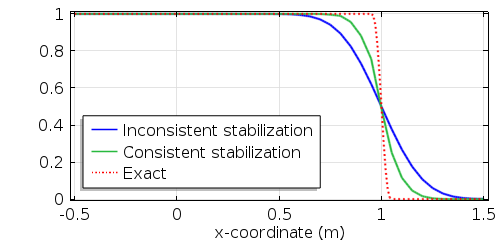This comparison for the concentration profile at t = 1 shows that for the same mesh (\Delta x=0.05), the inconsistent stabilization (blue line) introduces much more diffusion than the consistent stabilization (green line). This is the reason why the consistent stabilization is the default choice for all transport physics interfaces. That’s what we will discuss next.

#### Consistent Stabilization: Streamline and Crosswind Diffusion

Unlike the inconsistent method, the consistent method gives less numerical diffusion the closer the numerical solution comes to the exact solution. In other words, no diffusion is added in the regions where the mesh is fine enough.

Tip: You can read more about the mathematical details and references in the COMSOL Multiphysics Reference Manual.

The streamline diffusion method only adds diffusion in the streamline direction, which is also known as upwinding. As seen in the next plot, amplifying oscillations can usually be avoided by this method (blue line), but steep gradients can still lead to local disturbances (known as over- or undershoots). To overcome this problem, we also use crosswind diffusion.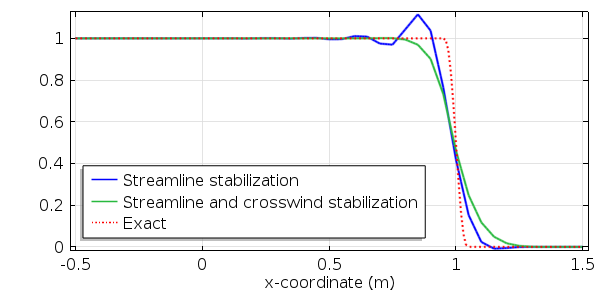Crosswind stabilization (green line) does not only add diffusion in the cross direction, it also captures discontinuities and, therefore, removes the numerical under- and overshoots. Consistent stabilization methods (streamline together with crosswind stabilization) are usually more efficient, because increasing the mesh resolution converges faster to the exact solution than inconsistent stabilization does. This, in turn, means that finding a physically acceptable solution requires less computational effort and time.

The previous plot shows that the consistent stabilization removes all oscillations and numerical over- or undershoots close to the sharp concentration gradients. The results are far from the analytic solution, though. This is due to the fact that the mesh is very coarse (\Delta x=0.05) compared to the size in the transition region in the initial concentration profile (\Delta transition=0.1) and the fact that first order elements are used. The next result shows that, as we refine the mesh, the solution converges to the exact one: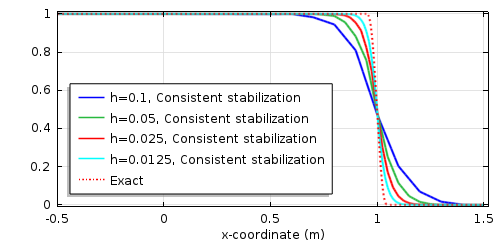The consistent stabilization methods guarantee that the problem is well resolved in space. Because we are solving a time-dependent problem, it is also a good idea to look at the time-dependent solver settings to make sure that the problem is also well resolved in time. In COMSOL Multiphysics, the accuracy of the time-dependent solver is controlled by the relative and absolute error tolerances.

The next plot shows the solution as a function of the user-defined error tolerances: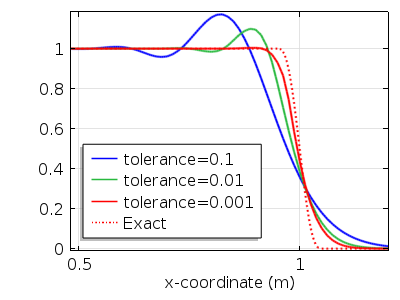A numerical overshoot is observed when the error tolerances are too loose and disappear for tolerances of 1e-3. Tighter tolerances lead to more accurate results, but increase the computational load. By default, the relative and absolute error tolerances are set to 1e-2 and 1e-3, respectively.

#### Physics-Specific Tips

##### 1. Transport of Diluted and Concentrated Species

The Transport of Diluted Species interface offers additional advanced options. One option is the internal calculation of the residual. In order to save time, it is most often not necessary to calculate the full residual for the current solution, but rather approximate it by neglecting the derivatives of the diffusion tensor components.Moreover, you can choose between two different crosswind diffusion methods: the “Do Carmo and Galeão” (default) and the “Codina” formulation. The first reduces over- and undershoots to a minimum and works also for anisotropic meshes. In case of convergence problems, even for optimal meshes, you should switch to the second formulation. The “Codina” formulation is less diffusive compared to the “Do Carmo and Galeão” option, but can result in more undershoots and overshoots.##### 2. Fluid Flow

The stabilization settings should, in general, not be modified in the fluid flow interfaces. The Navier-Stokes equations are unstable when using the default P1 + P1 elements independently of the Péclet number, and always require consistent stabilization (refer to the work of I. Babuška and F. Brezzi for more details). Pm + Pn means that the velocity is resolved with m order computational elements, while the pressure is resolved with n order elements.

The default consistent stabilization could only be removed when the velocity is resolved at a higher order than the pressure (P2 + P1 and P3 + P2) and when the Péclet number is small. These computational elements (P2 + P1 and P3 + P2) should only be used when the flow is well resolved by the mesh. In that case, the impact of the stabilization terms on the solution is negligible anyway and removing it won’t affect your results.

##### 3. Equation-Based Modeling

The physics interfaces in the COMSOL software include stabilization methods. If you are working with your own transport equations, however, you will need to do some literature review on stabilization methods for your particular application and implement the stabilization equations yourself. A first try can consist of adding artificial diffusion to your diffusive parameter manually. This example shows how to implement stabilization terms manually.

### Conclusion and Alternative Method

No need to change the default stabilization settings! Our developers, here at COMSOL, have done a fantastic job adding consistent artificial diffusion so that you don’t have to worry about stability issues. The consistent stabilization helps when the mesh is too coarse and hides when the equations are well resolved by the mesh. Be aware, however, that there is another way of solving mass transport problems without any artificial or viscous diffusion.

Yes, unlike grid-based methods (i.e., finite elements, finite differences, finite volumes), Lagrangian particle-based methods can very efficiently model high Péclet number problems without adding artificial diffusion. This alternative method is available in the Particle Tracing Module. COMSOL Certified Consultant, Veryst Engineering, collaborated with Nordson EFD to develop computational models of their laminar static mixers and found ways to improve and optimize their performance. Using COMSOL Multiphysics and the Particle Tracing Module, they were able to exclude numerical diffusion from the simulation for a more accurate solution, using far less computational resources than had they used grid-based methods.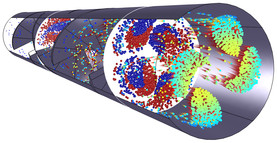To get you started with COMSOL Multiphysics, our Model Library includes the example of a static mixer solved with both methods (the grid-based transport of a diluted species physics and the particle tracing physics). Again, further details on numerical stabilization and the references can be found in the COMSOL Multiphysics Reference Manual.

#### Categories##### Joana Monteiro
July 7, 2014

Hi, I am solving a advection-diffusion equation with the transport of Diluted Species interface in comsol 4.3. I am adding crosswind and also isotropic diffusion in order to stabilize my solution. In the new version of comsol I think it is possible to choose the kind of crosswind stabilization technique we want (Do Carmo or Codina). However, in the 4.3 version we just can choose the value of the lower gradient limit, the default is 0.1. I would like to know what kind of crosswind diffusion comsol 4.3 uses. I could not find any information about it in the manual. Could you please give me any clue?
Thanks,
Joana.##### Fabrice Schlegel
July 14, 2014

Hi Joana,

Please submit this question to the technical support team at http://www.comsol.com/support.

Thanks,
Fabrice##### Aurelien Briffaz
September 26, 2014

How to use stabilization techniques through the PDE mode?

Best regards,
Aurelien Briffaz
Qualisud Research Unit, CIRAD, Montpellier, France##### Fabrice Schlegel
September 29, 2014

Hi Aurelien,

Please submit this question to the technical support team at http://www.comsol.com/support.

Thanks,
Fabrice##### Yoav Matia
July 6, 2016

Extremely informative, thank you very much.
One notable remark if i may, add a few more words on relative and absolute errors, This will help to better understand the dealing with the temporal approach.

Bravo.
Yoav.June 19, 2017

Hi,

This blog is very informative for my current project.
I have one simple question. In comsol, we only know maximum size and minimum size of the mesh. Then, which value is representing the h (mesh element size)?

Thank you,
Jalu##### Chien Liu
June 27, 2017

Dear Jalu,

Thank you for the comment. You can use the built-in variable “h” to evaluate the local mesh size.

Sincerely,
Chien##### Ali Davood
May 15, 2021

Hi, Thank you for this great article. I’ve read this multiple times but I can’t understand a point.

In “inconsistent stabilization”, we have an equation for artificial diffusion coefficient (c_art=delta*h*beta) . We can conclude from this equation that “c_art” and in turn, overall diffusion decreases when h decreases (or when we have fine mesh).

But somewhere in the article, about “consistent stabilization”, it is written that:
“Unlike the inconsistent method, the consistent method gives less numerical diffusion the closer the numerical solution comes to the exact solution. In other words, ((no diffusion is added in the regions where the mesh is fine enough)).”

I’m a little confused because both of the methods introduce less diffusion to the model when the mesh is fine, so there is no difference between them from this point of view, while the opposite is mentioned in the article. could you please inform me where I am misunderstanding?

EXPLORE COMSOL BLOG